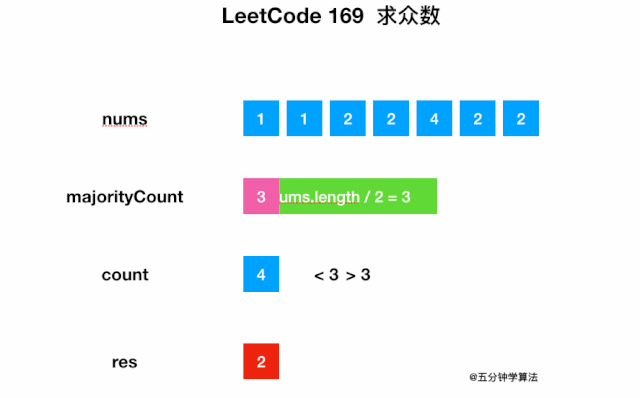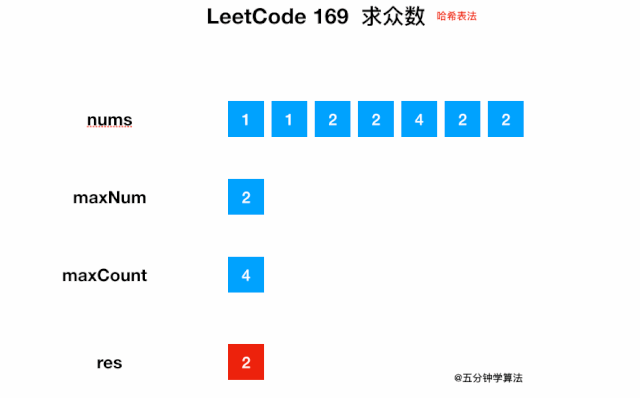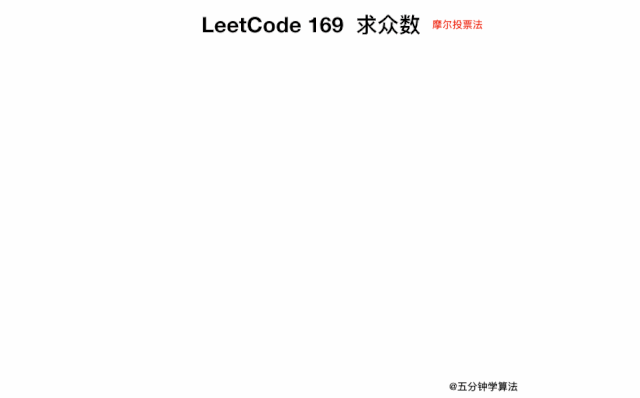输入: [3,2,3]输出: 3

输入: [2,2,1,1,1,2,2]输出: 2

### 动画描述### 代码实现

class Solution {    public int majorityElement(int[] nums) {        int majorityCount = nums.length/2;        for (int num : nums) {            int count = 0;            for (int elem : nums) {                if (elem == num) {                    count += 1;                }            }            if (count > majorityCount) {                return num;            }        }      }}

### 动画描述### 代码实现

class Solution {    public int majorityElement(int[] nums) {    Map<Integer, Integer> map = new HashMap<>();    // maxNum 表示元素，maxCount 表示元素出现的次数    int maxNum = 0, maxCount = 0;    for (int num: nums) {      int count = map.getOrDefault(num, 0) + 1;      map.put(num, count);      if (count > maxCount) {        maxCount = count;        maxNum = num;      }    }    return maxNum;  }}

### 复杂度分析

• 设置两个变量  candidate 和 count，candidate 用来保存数组中遍历到的某个数字，count 表示当前数字的出现次数，一开始 candidate 保存为数组中的第一个数字，count 为 1

• 遍历整个数组

• 如果数字与之前 candidate 保存的数字相同，则 count 加 1

• 如果数字与之前 candidate 保存的数字不同，则 count 减 1

• 如果出现次数 count 变为 0 ，candidate 进行变化，保存为当前遍历的那个数字，并且同时把 count 重置为 1

• 遍历完数组中的所有数字即可得到结果

### 动画描述### 代码实现

class Solution {    public int majorityElement(int[] nums) {    int candidate = nums, count = 1;    for (int i = 1; i < nums.length; ++i) {      if (count == 0) {        candidate = nums[i];        count = 1;      } else if (nums[i] == candidate) {        count++;      } else{        count--;      }    }    return candidate;  }}

### 复杂度分析

GitHub 标星 3w+，很全面的算法和数据结构知识